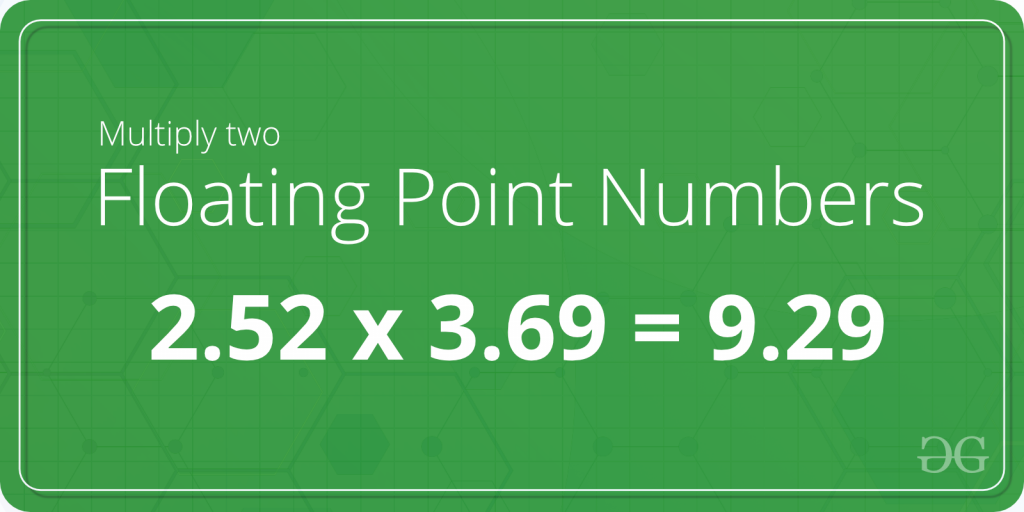# C Program to Multiply two Floating Point Numbers

Given two floating numbers A and B. The task is to write a program to find the product of these two numbers.Examples:

Input: A = 2.12, B = 3.88
Output: 8.225600

Input: A = 3.78, B = 6.32
Output: 23.889601

## Recommended: Please try your approach on {IDE} first, before moving on to the solution.

In the below program to multiply two floating point numbers, the user is first asked to enter two floating numbers and the input is scanned using the scanf() function and stored in the variablesand. Then, the variablesandare multiplied using the arithmetic operatorand the product is stored in the variable product.

Below is the C program to multiply two floating point numbers:

 `// C program to multiply two floating point numbers ` `#include ` ` `  `int` `main() ` `{ ` `    ``float` `A, B, product = 0.0f; ` ` `  `    ``// Ask user to enter the two numbers ` `    ``printf``(``"Enter two floating numbers A and B : \n"``); ` ` `  `    ``// Read two numbers from the user || A = 2.12, B = 3.88 ` `    ``scanf``(``"%f%f"``, &A, &B); ` ` `  `    ``// Calclulate the multiplication of A and B ` `    ``// using '*' operator ` `    ``product = A * B; ` ` `  `    ``// Print the product ` `    ``printf``(``"Product of A and B is: %f"``, product); ` ` `  `    ``return` `0; ` `} `

Output:

```Enter two floating numbers A and B : 2.12 3.88
Product of A and B is: 8.225600
```

Attention reader! Don’t stop learning now. Get hold of all the important DSA concepts with the DSA Self Paced Course at a student-friendly price and become industry ready.

My Personal Notes arrow_drop_upCheck out this Author's contributed articles.

If you like GeeksforGeeks and would like to contribute, you can also write an article using contribute.geeksforgeeks.org or mail your article to contribute@geeksforgeeks.org. See your article appearing on the GeeksforGeeks main page and help other Geeks.

Please Improve this article if you find anything incorrect by clicking on the "Improve Article" button below.

Article Tags :
Practice Tags :

Be the First to upvote.

Please write to us at contribute@geeksforgeeks.org to report any issue with the above content.